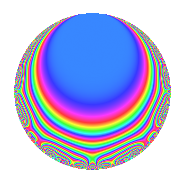# Properties

 Label 152.1.u.aLevel 152 Weight 1 Character orbit 152.u Analytic conductor 0.076 Analytic rank 0 Dimension 6 Projective image $$D_{9}$$ CM disc. -8 Inner twists 4

# Related objects

## Newspace parameters

 Level: $$N$$ = $$152 = 2^{3} \cdot 19$$ Weight: $$k$$ = $$1$$ Character orbit: $$[\chi]$$ = 152.u (of order $$18$$ and degree $$6$$)

## Newform invariants

 Self dual: No Analytic conductor: $$0.0758578819202$$ Analytic rank: $$0$$ Dimension: $$6$$ Coefficient field: $$\Q(\zeta_{18})$$ Coefficient ring: $$\Z[a_1, a_2]$$ Coefficient ring index: $$1$$ Projective image $$D_{9}$$ Projective field Galois closure of 9.1.69564674215936.1

## $q$-expansion

The $$q$$-expansion and trace form are shown below.

 $$f(q)$$ $$=$$ $$q -\zeta_{18}^{5} q^{2} + ( \zeta_{18}^{6} - \zeta_{18}^{7} ) q^{3} -\zeta_{18} q^{4} + ( \zeta_{18}^{2} - \zeta_{18}^{3} ) q^{6} + \zeta_{18}^{6} q^{8} + ( -\zeta_{18}^{3} + \zeta_{18}^{4} - \zeta_{18}^{5} ) q^{9} +O(q^{10})$$ $$q -\zeta_{18}^{5} q^{2} + ( \zeta_{18}^{6} - \zeta_{18}^{7} ) q^{3} -\zeta_{18} q^{4} + ( \zeta_{18}^{2} - \zeta_{18}^{3} ) q^{6} + \zeta_{18}^{6} q^{8} + ( -\zeta_{18}^{3} + \zeta_{18}^{4} - \zeta_{18}^{5} ) q^{9} + ( \zeta_{18}^{4} + \zeta_{18}^{8} ) q^{11} + ( -\zeta_{18}^{7} + \zeta_{18}^{8} ) q^{12} + \zeta_{18}^{2} q^{16} + \zeta_{18}^{5} q^{17} + ( 1 - \zeta_{18} + \zeta_{18}^{8} ) q^{18} -\zeta_{18} q^{19} + ( 1 + \zeta_{18}^{4} ) q^{22} + ( -\zeta_{18}^{3} + \zeta_{18}^{4} ) q^{24} -\zeta_{18}^{7} q^{25} + ( 1 - \zeta_{18} + \zeta_{18}^{2} - \zeta_{18}^{3} ) q^{27} -\zeta_{18}^{7} q^{32} + ( -\zeta_{18} + \zeta_{18}^{2} - \zeta_{18}^{5} + \zeta_{18}^{6} ) q^{33} + \zeta_{18} q^{34} + ( \zeta_{18}^{4} - \zeta_{18}^{5} + \zeta_{18}^{6} ) q^{36} + \zeta_{18}^{6} q^{38} + ( -\zeta_{18} - \zeta_{18}^{3} ) q^{41} -\zeta_{18}^{8} q^{43} + ( 1 - \zeta_{18}^{5} ) q^{44} + ( 1 + \zeta_{18}^{8} ) q^{48} + \zeta_{18}^{6} q^{49} -\zeta_{18}^{3} q^{50} + ( -\zeta_{18}^{2} + \zeta_{18}^{3} ) q^{51} + ( -\zeta_{18}^{5} + \zeta_{18}^{6} - \zeta_{18}^{7} + \zeta_{18}^{8} ) q^{54} + ( -\zeta_{18}^{7} + \zeta_{18}^{8} ) q^{57} + ( -\zeta_{18}^{3} - \zeta_{18}^{7} ) q^{59} -\zeta_{18}^{3} q^{64} + ( -\zeta_{18} + \zeta_{18}^{2} + \zeta_{18}^{6} - \zeta_{18}^{7} ) q^{66} + ( -\zeta_{18}^{3} - \zeta_{18}^{5} ) q^{67} -\zeta_{18}^{6} q^{68} + ( 1 - \zeta_{18} + \zeta_{18}^{2} ) q^{72} + ( 1 + \zeta_{18}^{4} ) q^{73} + ( \zeta_{18}^{4} - \zeta_{18}^{5} ) q^{75} + \zeta_{18}^{2} q^{76} + ( 1 - \zeta_{18} + \zeta_{18}^{6} - \zeta_{18}^{7} + \zeta_{18}^{8} ) q^{81} + ( \zeta_{18}^{6} + \zeta_{18}^{8} ) q^{82} + ( \zeta_{18}^{2} + \zeta_{18}^{4} ) q^{83} -\zeta_{18}^{4} q^{86} + ( -\zeta_{18} - \zeta_{18}^{5} ) q^{88} + \zeta_{18}^{7} q^{89} + ( \zeta_{18}^{4} - \zeta_{18}^{5} ) q^{96} + ( \zeta_{18}^{4} + \zeta_{18}^{6} ) q^{97} + \zeta_{18}^{2} q^{98} + ( 1 + \zeta_{18}^{2} - \zeta_{18}^{3} + \zeta_{18}^{4} - \zeta_{18}^{7} + \zeta_{18}^{8} ) q^{99} +O(q^{100})$$ $$\operatorname{Tr}(f)(q)$$ $$=$$ $$6q - 3q^{3} - 3q^{6} - 3q^{8} - 3q^{9} + O(q^{10})$$ $$6q - 3q^{3} - 3q^{6} - 3q^{8} - 3q^{9} + 6q^{18} + 6q^{22} - 3q^{24} + 3q^{27} - 3q^{33} - 3q^{36} - 3q^{38} - 3q^{41} + 6q^{44} + 6q^{48} - 3q^{49} - 3q^{50} + 3q^{51} - 3q^{54} - 3q^{59} - 3q^{64} - 3q^{66} - 3q^{67} + 3q^{68} + 6q^{72} + 6q^{73} + 3q^{81} - 3q^{82} - 3q^{97} + 3q^{99} + O(q^{100})$$

## Character Values

We give the values of $$\chi$$ on generators for $$\left(\mathbb{Z}/152\mathbb{Z}\right)^\times$$.

 $$n$$ $$39$$ $$77$$ $$97$$ $$\chi(n)$$ $$-1$$ $$-1$$ $$-\zeta_{18}$$

## Embeddings

For each embedding $$\iota_m$$ of the coefficient field, the values $$\iota_m(a_n)$$ are shown below.

For more information on an embedded modular form you can click on its label.

Label $$\iota_m(\nu)$$ $$a_{2}$$ $$a_{3}$$ $$a_{4}$$ $$a_{5}$$ $$a_{6}$$ $$a_{7}$$ $$a_{8}$$ $$a_{9}$$ $$a_{10}$$
35.1
 −0.173648 − 0.984808i −0.766044 + 0.642788i −0.766044 − 0.642788i 0.939693 − 0.342020i 0.939693 + 0.342020i −0.173648 + 0.984808i
0.766044 + 0.642788i −1.43969 + 0.524005i 0.173648 + 0.984808i 0 −1.43969 0.524005i 0 −0.500000 + 0.866025i 1.03209 0.866025i 0
43.1 −0.939693 + 0.342020i −0.326352 + 1.85083i 0.766044 0.642788i 0 −0.326352 1.85083i 0 −0.500000 + 0.866025i −2.37939 0.866025i 0
99.1 −0.939693 0.342020i −0.326352 1.85083i 0.766044 + 0.642788i 0 −0.326352 + 1.85083i 0 −0.500000 0.866025i −2.37939 + 0.866025i 0
123.1 0.173648 + 0.984808i 0.266044 0.223238i −0.939693 + 0.342020i 0 0.266044 + 0.223238i 0 −0.500000 0.866025i −0.152704 + 0.866025i 0
131.1 0.173648 0.984808i 0.266044 + 0.223238i −0.939693 0.342020i 0 0.266044 0.223238i 0 −0.500000 + 0.866025i −0.152704 0.866025i 0
139.1 0.766044 0.642788i −1.43969 0.524005i 0.173648 0.984808i 0 −1.43969 + 0.524005i 0 −0.500000 0.866025i 1.03209 + 0.866025i 0
 $$n$$: e.g. 2-40 or 990-1000 Embeddings: e.g. 1-3 or 139.1 Significant digits: Format: Complex embeddings Normalized embeddings Satake parameters Satake angles

## Inner twists

Char. orbit Parity Mult. Self Twist Proved
1.a Even 1 trivial yes
8.d Odd 1 CM by $$\Q(\sqrt{-2})$$ yes
19.e Even 1 yes
152.u Odd 1 yes

## Hecke kernels

There are no other newforms in $$S_{1}^{\mathrm{new}}(152, [\chi])$$.